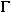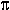DOC HOME SITE MAP MAN PAGES GNU INFO SEARCH PRINT BOOK
C compilation system

# Math library (libm)

The math library, libm, contains the mathematics functions supplied by the C compilation system. This section describes some of the major functions,
organized by the manual page on which they appear. Note that functions whose names end with the letter ``f'' are single-precision versions, which means that their argument and return types are float. Functions whose names end with the letter ``l'' are long double-precision versions, which means that their argument and return types are long double. The header file math.h should be included in programs that use math functions.

Math functions

exp(S)
exp expf   Return e^x.
cbrt     Return cube root ofx.
log logf   Return the natural logarithm of x. The value of x must be positive.
log10 log10f   Return the base-ten logarithm of x. The value of x must be positive.
pow powf   Return x^y. If x is zero, y must be positive. If x is negative, y must be an integer.
sqrt sqrtf   Return the non-negative square root of x. The value of x must be non-negative.
hypot(S)
hypot     Return sqrt(xx + yy), taking precautions against overflows.
gamma(S)
gamma lgamma   Return ln(|(x)| ), where(x) is defined as \$int from 0 to x e sup -t t sup x-1 dt\$.
trig(S)
sin sinf   Return, respectively, the sine, cosine, and tangent of x, measured in radians.
cos cosf
tan tanf
asin asinf   Return the arcsine of x, in the range [-/2, +/2].
acos acosf   Return the arccosine of x, in the range [0,+].
atan atanf   Return the arctangent of x, in the range (-/2, +/2).
atan2 atan2f   Return the arctangent of y/x, in the range (-, +], using the signs of both arguments to determine the quadrant of the return value.
sinh(S)
sinh sinhf   Return, respectively, the hyperbolic sine, cosine, and tangent of their argument.
cosh coshf
tanh tanhf
asinh acosh atanh     Return, respectively, the inverse hyperbolic sine, cosine, and tangent of their argument.
matherr(S)
matherr     Error handling.
erf(S)
erf     Returns the error function of x, defined as \$ 2 over sqrt pi int from 0 to x e sup {-t sup 2}dt\$.
erfc     erfc, which returns 1.0 - erf(x), is provided because of the extreme loss of relative accuracy if erf is called for large x and the result subtracted from 1.0 (for example, for x = 5, 12 places are lost).
floor(S)
floor floorf   Return the largest integer not greater than x.
ceil ceilf   Return the smallest integer not less than x.
copysign     Return x but with the sign of y.
fmod fmodf   Return the floating point remainder of the division of x by y: x if y is zero, otherwise the number f with same sign as x, such that x = iy + f for some integer i, and | f |<| y |.
fabs fabsf   Return the absolute value of x, | x |.
rint     Return the integer value nearest to the double-precision floating point argument x as a double-precision floating point number. The returned value is rounded according to the currently set machine rounding mode. If round-to-nearest (the default mode) is set and the difference between the function argument and the rounded result is exactly 0.5, then the result will be rounded to the nearest even integer.
remainder     Return the floating point remainder of the division of x by y: NaN if y is zero, otherwise the value r = x - yn, where n is the integer nearest the exact value of x. Whenever |n - x/y| = 1/2, then n is even.

Next topic: General purpose library (libgen)
Previous topic: System calls